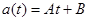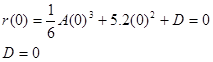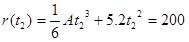# 03. Using the Calculus Another Example

$$\newcommand{\vecs}{\overset { \rightharpoonup} {\mathbf{#1}} }$$ $$\newcommand{\vecd}{\overset{-\!-\!\rightharpoonup}{\vphantom{a}\smash {#1}}}$$$$\newcommand{\id}{\mathrm{id}}$$ $$\newcommand{\Span}{\mathrm{span}}$$ $$\newcommand{\kernel}{\mathrm{null}\,}$$ $$\newcommand{\range}{\mathrm{range}\,}$$ $$\newcommand{\RealPart}{\mathrm{Re}}$$ $$\newcommand{\ImaginaryPart}{\mathrm{Im}}$$ $$\newcommand{\Argument}{\mathrm{Arg}}$$ $$\newcommand{\norm}{\| #1 \|}$$ $$\newcommand{\inner}{\langle #1, #2 \rangle}$$ $$\newcommand{\Span}{\mathrm{span}}$$ $$\newcommand{\id}{\mathrm{id}}$$ $$\newcommand{\Span}{\mathrm{span}}$$ $$\newcommand{\kernel}{\mathrm{null}\,}$$ $$\newcommand{\range}{\mathrm{range}\,}$$ $$\newcommand{\RealPart}{\mathrm{Re}}$$ $$\newcommand{\ImaginaryPart}{\mathrm{Im}}$$ $$\newcommand{\Argument}{\mathrm{Arg}}$$ $$\newcommand{\norm}{\| #1 \|}$$ $$\newcommand{\inner}{\langle #1, #2 \rangle}$$ $$\newcommand{\Span}{\mathrm{span}}$$$$\newcommand{\AA}{\unicode[.8,0]{x212B}}$$

# Another Example

Investigate the scenario described below.

A sports car can accelerate from rest to a speed of 40 m/s while traveling a distance of 200 m. Assume the acceleration of the car can be modeled as a decreasing linear function of time, with a maximum acceleration of 10.4 m/s2.Between event 1 and 2, the car's acceleration can be modeled by a generic linear function of time, orSince the acceleration is decreasing, the maximum value occurs at t = 0 s,Since we don’t know the value of the acceleration at t2, or even the value of t2, we can’t determine A, and all we can currently say about the acceleration function is that it is given by:Nonetheless, we can still integrate the acceleration to determine the velocity,Since we know v = 0 m/s when t = 0 s, we can determine the integration constant:We also know that v = 40 m/s at t2, so:This equation can’t be solved, since it involves two unknowns. However, if we can generate a second equation involving the same two unknowns, we can solve the two equations simultaneously. This second equation must involve the position function of the car:Since we know r = 0 when t = 0 s, we can determine the integration constant:We also know that r = 200 m at t2, so:These two equations,can be solved by substitution (or by using a solver). Solve the first equation for A, and substitute this expression into the second equation. This will result in a quadratic equation for t2. The solution is t2 = 7.57 s, the time for the car to reach 40 m/s. Plugging this value back into the original equations allows you to complete the description of the car’s motion.

This page titled 03. Using the Calculus Another Example is shared under a CC BY-NC-SA license and was authored, remixed, and/or curated by Paul D'Alessandris.